# Excel Absolute References

## Absolute References

Absolute reference is when a reference has the dollar sign (`\$`).

It locks a reference in the formula.

Add `\$` to the formula to use absolute references.

The dollar sign has three different states:

• Absolute for column and row. The reference is absolutely locked.
Example `=\$A\$1`

• Absolute for the column. The reference is locked to that column. The row remains relative.
Example `=\$A1`

• Absolute for the row. The reference is locked to that row. The column remains relative.
Example `=A\$1`

Let's have a look at an example helping the Pokemon trainers to calculate prices for Pokeballs

Type or copy the following data: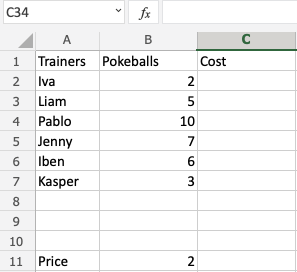Copy Values

Data explained

• There are 6 trainers: Iva, Liam, Adora, Jenny, Iben and Kasper.
• They have different amount of Pokeballs each in their shop cart
• The price per Pokeball is 2 coins

Help them to calculate the prices for the Pokeballs.

The price's reference is `B11`, we do not want the fill function to change this, so we lock it.

The reference is absolutely locked by using the formula `\$B\$11`.

How to do it, step by step:

1. Type `C2(=)`
2. Select `B11`
3. Type (`\$`) before the `B` and `11` (`\$B\$11`)
4. Type (`*`)
5. Select `B2`
6. Hit enter
7. Auto fill `C2:C7`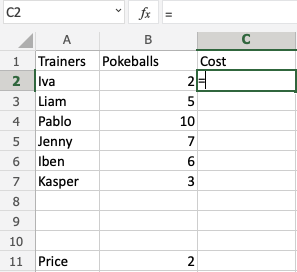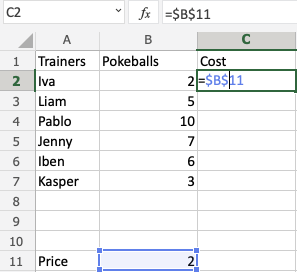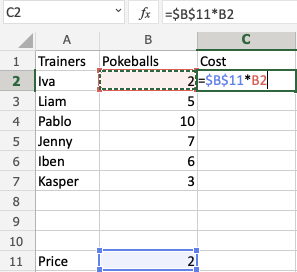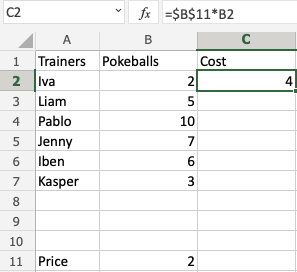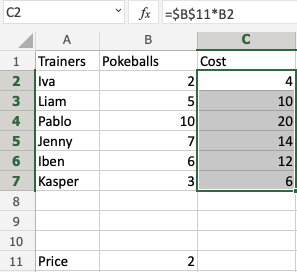Congratulations! You successfully calculated the prices for the Pokeballs using an absolute reference.

## Excel Exercise:

Type reference `A1` as absolute:

```A\$1
```

Start the Exercise